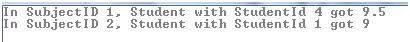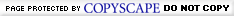# Select the Highest value in each Group or Category using LINQ

Posted by: Suprotim Agarwal , on 11/12/2009, in Category LINQ
Views: 57611
Abstract: In this article, we will see how to select the highest value in a Group or Category using LINQ
Select the Highest value in each Group or Category using LINQ

Sometime back I had written an article on Selecting the Highest value in a Group/Category using SQL Server 2005/2008. A user mailed back asking how to use LINQ to find the highest value in a group/category. This article shows you how to do so.
We will be using the Enumerable.Max method in this example that returns the maximum value in a sequence of values.
To start with, we will use a Student class for our example with the following definition:
C#
class Students
{
public int StudentId {get; set;}
public int SubjectId {get; set;}
public float Marks { get; set; }
}
VB.NET
Class Students

Private privateStudentId As Integer
Public Property StudentId() As Integer
Get
Return privateStudentId
End Get
Set(ByVal value As Integer)
privateStudentId = value
End Set
End Property

Private privateSubjectId As Integer
Public Property SubjectId() As Integer
Get
Return privateSubjectId
End Get
Set(ByVal value As Integer)
privateSubjectId = value
End Set
End Property

Private privateMarks As Single
Public Property Marks() As Single
Get
Return privateMarks
End Get
Set(ByVal value As Single)
privateMarks = value
End Set
End Property

End Class
Our task is to list the Student with the highest mark in a Subject. To do so, we will write the following code:
C#
class Program
{
static void Main(string[] args)
{
List<Students> students = new List<Students>();
students.Add(new Students() { StudentId = 1, SubjectId = 1, Marks = 8.0f });
students.Add(new Students() { StudentId = 2, SubjectId = 1, Marks = 5.0f });
students.Add(new Students() { StudentId = 3, SubjectId = 1, Marks = 7.0f });
students.Add(new Students() { StudentId = 4, SubjectId = 1, Marks = 9.5f });
students.Add(new Students() { StudentId = 1, SubjectId = 2, Marks = 9.0f });
students.Add(new Students() { StudentId = 2, SubjectId = 2, Marks = 7.0f });
students.Add(new Students() { StudentId = 3, SubjectId = 2, Marks = 4.0f });
students.Add(new Students() { StudentId = 4, SubjectId = 2, Marks = 7.5f });

var stud = from s in students
group s by s.SubjectId into stugrp
let topp = stugrp.Max(x => x.Marks)
select new
{
Subject = stugrp.Key,
TopStudent = stugrp.First(y => y.Marks == topp).StudentId,
MaximumMarks = topp
};
}
}
VB.NET
Sub Main()
Dim students As New List(Of Students)()
students.Add(New Students() With {.StudentId = 1, .SubjectId = 1, .Marks = 8.0F})
students.Add(New Students() With {.StudentId = 2, .SubjectId = 1, .Marks = 5.0F})
students.Add(New Students() With {.StudentId = 3, .SubjectId = 1, .Marks = 7.0F})
students.Add(New Students() With {.StudentId = 4, .SubjectId = 1, .Marks = 9.5F})
students.Add(New Students() With {.StudentId = 1, .SubjectId = 2, .Marks = 9.0F})
students.Add(New Students() With {.StudentId = 2, .SubjectId = 2, .Marks = 7.0F})
students.Add(New Students() With {.StudentId = 3, .SubjectId = 2, .Marks = 4.0F})
students.Add(New Students() With {.StudentId = 4, .SubjectId = 2, .Marks = 7.5F})

Dim stud = _
From s In students _
Group s By Key = s.SubjectId Into Group _
Let topp = Group.Max(Function(x) x.Marks) _
Select New With {Key .Subject = Key, _
Key .TopStudent = Group.First(Function(y) y.Marks = topp).StudentId, _
Key .MaximumMarks = topp}

End Sub
The implementation shown here is quite simple (thanks to Drew M). We first select the maximum marks in a subject using
let topp = stugrp.Max(x => x.Marks)
and then use the same ‘topp’ variable to compare it against the score of a student in that subject and then select the studentid
TopStudent = stugrp.First(y => y.Marks == topp).StudentId
That’s it. The final step is to loop through the results and print the values
C#
foreach (var student in stud)
{
Console.WriteLine("In SubjectID {0}, Student with StudentId {1} got {2}",
student.Subject,
student.TopStudent,
student.MaximumMarks);
}

VB.NET
For Each student In stud
Console.WriteLine("In SubjectID {0}, Student with StudentId {1} got {2}", _  student.Subject, student.TopStudent, student.MaximumMarks)
Next student

The output is as shown below:C# and .NET have been around for a very long time, but their constant growth means there’s always more to learn.

We at DotNetCurry are very excited to announce The Absolutely Awesome Book on C# and .NET. This is a 500 pages concise technical eBook available in PDF, ePub (iPad), and Mobi (Kindle).

Organized around concepts, this Book aims to provide a concise, yet solid foundation in C# and .NET, covering C# 6.0, C# 7.0 and .NET Core, with chapters on the latest .NET Core 3.0, .NET Standard and C# 8.0 (final release) too. Use these concepts to deepen your existing knowledge of C# and .NET, to have a solid grasp of the latest in C# and .NET OR to crack your next .NET Interview.

AuthorSuprotim Agarwal, MCSD, MCAD, MCDBA, MCSE, is the founder of DotNetCurry, DNC Magazine for Developers, SQLServerCurry and DevCurry. He has also authored a couple of books 51 Recipes using jQuery with ASP.NET Controls and The Absolutely Awesome jQuery CookBook.

Suprotim has received the prestigious Microsoft MVP award for Fifteen consecutive years. In a professional capacity, he is the CEO of A2Z Knowledge Visuals Pvt Ltd, a digital group that offers Digital Marketing and Branding services to businesses, both in a start-up and enterprise environment.Feedback - Leave us some adulation, criticism and everything in between!
 Comment posted by Geoff on Thursday, November 12, 2009 7:56 AM
The link to go to page 2 is broken in google chrome as is the Insert button for this comment...
 Comment posted by Admin on Thursday, November 12, 2009 9:13 PM
@Geoff - We just tried replication the behavior in Chrome 3.0.195..works just fine..can you tell which version of Chrome are you using.
 Comment posted by Gaurav Dixit on Friday, November 13, 2009 12:38 AM
 Comment posted by Tadas on Saturday, November 14, 2009 7:15 PM
It won't work if there is few students with same Marks in the same group